# W3cubDocs

/Qt 5.11

The QGradient class is used in combination with QBrush to specify gradient fills. More...

 Header: #include qmake: QT += gui Inherited By:

## Detailed Description

The QGradient class is used in combination with QBrush to specify gradient fills.

Qt currently supports three types of gradient fills:

• Linear gradients interpolate colors between start and end points.
• Simple radial gradients interpolate colors between a focal point and end points on a circle surrounding it.
• Extended radial gradients interpolate colors between a center and a focal circle.
• Conical gradients interpolate colors around a center point.

A gradient's type can be retrieved using the type() function. Each of the types is represented by a subclass of QGradient: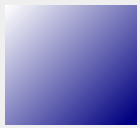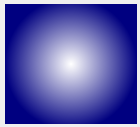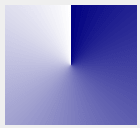The colors in a gradient are defined using stop points of the QGradientStop type; i.e., a position and a color. Use the setColorAt() function to define a single stop point. Alternatively, use the setStops() function to define several stop points in one go. Note that the latter function replaces the current set of stop points.

It is the gradient's complete set of stop points (accessible through the stops() function) that describes how the gradient area should be filled. If no stop points have been specified, a gradient of black at 0 to white at 1 is used.

A diagonal linear gradient from black at (100, 100) to white at (200, 200) could be specified like this:

```    QLinearGradient linearGrad(QPointF(100, 100), QPointF(200, 200));

A gradient can have an arbitrary number of stop points. The following would create a radial gradient starting with red in the center, blue and then green on the edges:

```    QRadialGradient radialGrad(QPointF(100, 100), 100);

It is possible to repeat or reflect the gradient outside its area by specifiying the spread method using the setSpread() function. The default is to pad the outside area with the color at the closest stop point. The currently set spread method can be retrieved using the spread() function. The QGradient::Spread enum defines three different methods: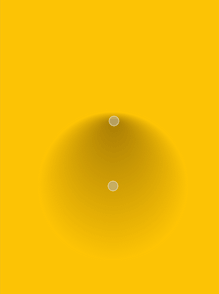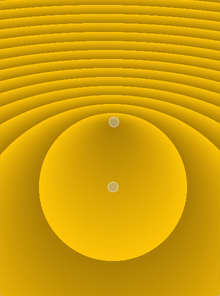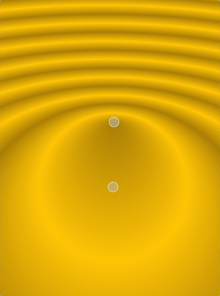PadSpread RepeatSpread ReflectSpread

Note that the setSpread() function only has effect for linear and radial gradients. The reason is that the conical gradient is closed by definition, i.e. the conical gradient fills the entire circle from 0 - 360 degrees, while the boundary of a radial or a linear gradient can be specified through its radius or final stop points, respectively.

The gradient coordinates can be specified in logical coordinates, relative to device coordinates, or relative to object bounding box coordinates. The coordinate mode can be set using the setCoordinateMode() function. The default is LogicalMode, where the gradient coordinates are specified in the same way as the object coordinates. To retrieve the currently set coordinate mode use coordinateMode().

## Member Type Documentation

This enum specifies how gradient coordinates map to the paint device on which the gradient is used.

Constant Value Description
`QGradient::LogicalMode` `0` This is the default mode. The gradient coordinates are specified logical space just like the object coordinates.
`QGradient::StretchToDeviceMode` `1` In this mode the gradient coordinates are relative to the bounding rectangle of the paint device, with (0,0) in the top left corner, and (1,1) in the bottom right corner of the paint device.
`QGradient::ObjectBoundingMode` `2` In this mode the gradient coordinates are relative to the bounding rectangle of the object being drawn, with (0,0) in the top left corner, and (1,1) in the bottom right corner of the object's bounding rectangle.

This enum was introduced or modified in Qt 4.4.

Specifies how the area outside the gradient area should be filled.

Constant Value Description
`QGradient::PadSpread` `0` The area is filled with the closest stop color. This is the default.
`QGradient::RepeatSpread` `2` The gradient is repeated outside the gradient area.
`QGradient::ReflectSpread` `1` The gradient is reflected outside the gradient area.

Specifies the type of gradient.

Constant Value Description
`QGradient::LinearGradient` `0` Interpolates colors between start and end points (QLinearGradient).
`QGradient::RadialGradient` `1` Interpolate colors between a focal point and end points on a circle surrounding it (QRadialGradient).
`QGradient::ConicalGradient` `2` Interpolate colors around a center point (QConicalGradient).
`QGradient::NoGradient` `3` No gradient is used.

## Member Function Documentation

Returns the coordinate mode of this gradient. The default mode is LogicalMode.

This function was introduced in Qt 4.4.

### void QGradient::setColorAt(qrealposition, const QColor &color)

Creates a stop point at the given position with the given color. The given position must be in the range 0 to 1.

Sets the coordinate mode of this gradient to mode. The default mode is LogicalMode.

This function was introduced in Qt 4.4.

Specifies the spread method that should be used for this gradient.

Note that this function only has effect for linear and radial gradients.

Replaces the current set of stop points with the given stopPoints. The positions of the points must be in the range 0 to 1, and must be sorted with the lowest point first.

Returns the stop points for this gradient.

If no stop points have been specified, a gradient of black at 0 to white at 1 is used.

Returns the type of gradient.

Returns `true` if the gradient is the same as the other gradient specified; otherwise returns `false`.

This function was introduced in Qt 4.2.

Returns `true` if the gradient is the same as the other gradient specified; otherwise returns `false`.